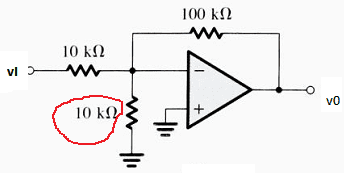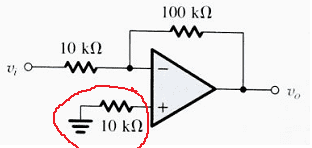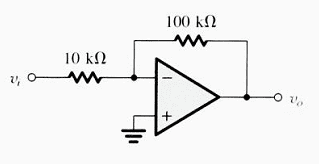# Find voltage gain and input resistance for op-amp

## Homework Statement

Find the input resistances and voltage gains for those ideal op amps## The Attempt at a Solution

The original inverting circuit look like this :we already have the equations :
input resistance = 10k
voltage gain = -r2/r1 = -10
For the first circuit :
it still a inverting op amps, does the red marked 10k resistor get involved with input resistances ? I think it's not because it connected to the ground (virtual ?). R2 is 100k so the equation for voltage gain remains the same as the original circuit .
Second circuit:
there is no current in red marked 10k resistor, input resistance is unchanged (10k), voltage gain remains (-10k)

I find it is difficult to calculate using op amps characteristics, can I use voltage node method to find the voltage gain, which node should I choose. Are those ground connected resistor have no effect on the circuit input resistances and voltage gain at all ?

berkeman
Mentor
You are correct that because of the virtual ground property (especially with ideal opamps), the input resistances of these circuits is 10kOhms. You have a typo in the gains, however. Can you see what your typo is?

You are correct that because of the virtual ground property (especially with ideal opamps), the input resistances of these circuits is 10kOhms. You have a typo in the gains, however. Can you see what your typo is?

Yes, for the second circuit the gain is -10.

So can we say in those cases : In an inverting op-amp, any resistors connected to the ground can be ignored when calculate ?

berkeman
Mentor
So can we say in those cases : In an inverting op-amp, any resistors connected to the ground can be ignored when calculate ?

I wouldn't say that -- it's a bit too simplified and not always true. Instead, understand what the "virtual ground" means. Can you tell us what is going on with the "virtual ground" property of a high-gain opamp with negative feedback means?